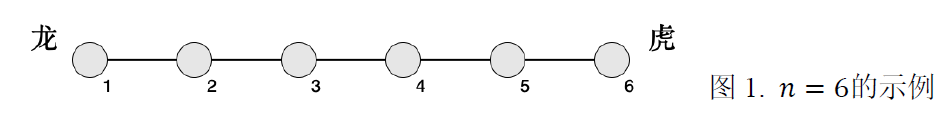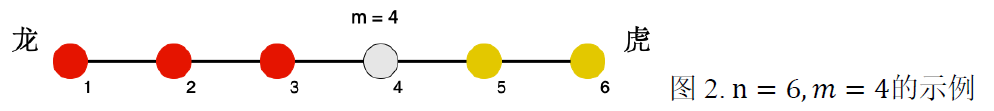# Description# Sample

## Input1

``````6
2 3 2 3 2 3
4 6 5 2
``````

## Output1

``````2
``````

## Hint1

\[2 × (4 − 1) + 3 × (4 − 2) + 2 × (4 − 3) = 14\]

\[2 × (5 − 4) + (3 + 5) × (6 − 4) = 18\]

\[14 + 2 × (4 − 2) = 18\]

## Input2

``````6
1 1 1 1 1 16
5 4 1 1
``````

## Output2

``````1
``````

## Hint2

\[1 × (5 − 1) + 1 × (5 − 2) + 1 × (5 − 3) + (1 + 1) × (5 − 4) = 11\]

\[16 × (6 − 5) = 16\]

\[11 + 1 × (5 − 1) = 15\]

# Limitation

\(1 < 𝑚 < 𝑛, 1 ≤ 𝑝_1 ≤ 𝑛\)。

NOIP2018普及组第二题

ID
1002

2

(无)

8

3

38%

NOIP普及组